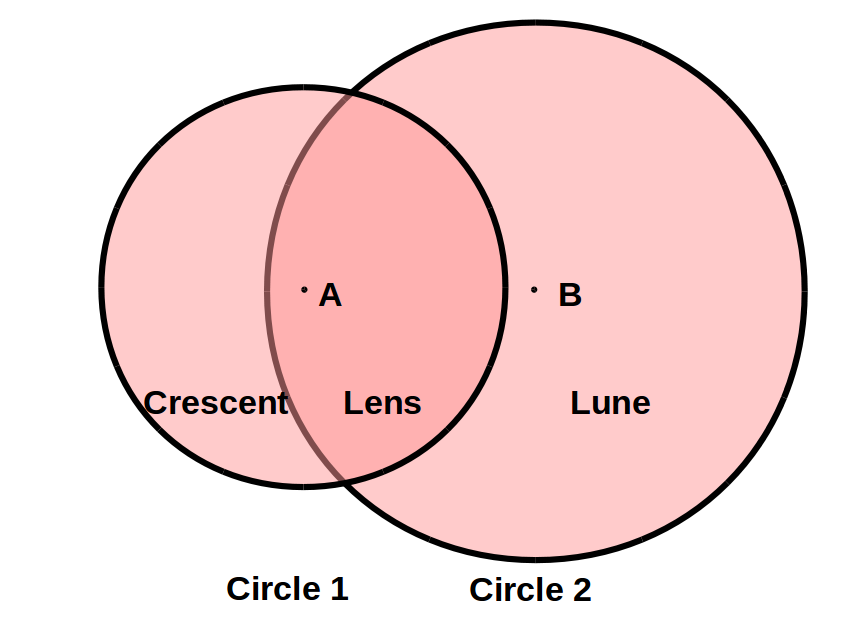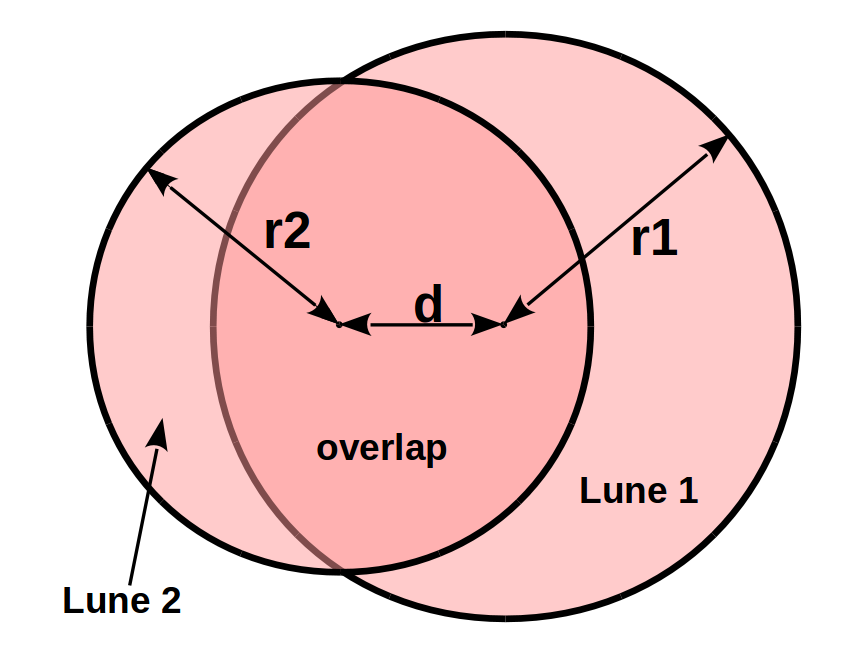# Area of Crescent Calculator

Created by Philip Maus
Reviewed by Hanna Pamuła, PhD and Jack Bowater
Last updated: Dec 13, 2022

The area of crescent calculator allows you to find the area for a geometrical shape consisting of two circular arcs. It points out what a crescent is, the differences between crescent, lune, and lens, and how to calculate the area of a crescent shape and lune shape.

## What is a crescent?

Geometrical regions consisting of two circular arcs separate into two groups:• A convex-convex region, in which both arcs curve outwards, is called a lens; and
• A concave-convex region, in which one arc curves outwards and one arc is curved inwards, is called a lune. You can also understand this geometrical shape as one disk partly covering another disk.

So, what is a crescent? A crescent is a lune which does not include the original disk's center. It means that the distance between the disk centers is smaller than the radius of the covering disk.

## Crescents in history

But a crescent is so much more than a geometric shape.

What else is a crescent? Where else do crescent-shaped things show up?

Its name comes from the Latin verb crescere, which means to grow and refers to the first quarter of the moon's cycle. The crescent shape is often used as a symbol for the moon, with examples dating back as far as Ancient Egypt. It can represent virginity and chastity, standing for the Virgin Mary in Christianity.

The crescent combined with a star dates back to the Sumerian civilization, with the crescent representing the moon and the star representing Venus. This symbol survived the centuries, becoming the symbol of the Ottoman Empire, who's influence caused it to become a symbol for Islam, present in the flags of numerous countries, namely Turkey, Azerbaijan, Pakistan, and Malaysia.

## Area of crescent formula

Let's turn away from the symbolic mysteries of crescents and investigate them mathematically.

A circle offers a plethora of exciting formulas and geometrical concepts. Starting with easy calculations about the area of a circle and its circumference, one soon ends up digging deeper with topics like the semicircle area, sector area, segment area, and the length of arcs. Let's expand our circle knowledge by the calculation of the area of a lune and a crescent.The image above displays two overlapping circles. Marked are r1 and r2, the radii of the circles 1 and 2 respectively, and the distance d between the circle's centers. The circle's arcs form the lune areas 1 and 2 and the overlapping area.

The area for the lune of circle 1 is can be computed as:

\scriptsize \begin{aligned} &\text{lune}_1 = \\ & \frac{1}{2} \sqrt{ (r_1\!\!+\!\!r_2\!\!+\!\!d)(r_2\!\!+\!\!d\!\!-\!\!r_1)(d\!\!+\!\!r_1\!\!-\!\!r_2)(r_1\!\!+\!\!r_2\!\!-\!\!d) } \\ & + r_1^2 \arccos\left( \frac{r_2^2-r_1^2-d^2}{2\cdot r_1\cdot d} \right) \\ & - r_2^2 \arccos\left( \frac{r_2^2+d^2-r_1^2}{2\cdot r_2\cdot d} \right) \\ \end{aligned}

Knowing the area of the lune, we can calculate the overlapping area of the two circles by subtracting the lune from the total area of circle 1:

$\scriptsize \text{overlap area} = \pi r_1^2 - \text{lune}_1$

And since the second circle consists of the second lune and the common overlap area, we calculate the area of the lune of circle 2 with the same formula:

$\scriptsize \text{lune}_2 = \pi r_2^2 - \text{overlap area}$

The source for the formulas above is .

## How to use the area of crescent calculator?

Now that you know what a crescent is and how to calculate the area of a crescent shape and the area of a lune let us take a closer look at how to use the area of crescent calculator. Remember that a crescent is a particular type of lune, in which the geometrical region does not include the center of the original circle.

1. Decide on a radius for circle 1. Let's assume it is 4 cm.
2. Now define the radius of circle 2. As an example, we will use 6 cm.
3. Define the distance between the two circle centers. It will determine which type of geometrical shape will form.
• By definition, the distance has to be bigger than the difference between the two radii, but smaller than the smaller circle's diameter. Otherwise, the circles do not overlap, and so no lune forms.
• For the lune to become a crescent, the distance between the two circle centers has to be smaller than the other circle's radius.

Let's use a distance of 5 cm. As explained, with this setting, lune 1 is a crescent, but lune 2 is not.

1. The lune/crescent areas and the overlapping area are computed according to the formulas mentioned above. The results are:
• Area of lune 1: 20.96 cm²;
• Area of lune 2: 83.70 cm²;
• Overlap area: 29.30 cm²;
• Lune 1 is a crescent; and
• Lune 2 is not a crescent.

Now you know what a crescent is, you learned a lot about crescent-shaped things in history and today's world, and you know how to calculate the area of a crescent shape.

Philip Mausin
in
Circle center distance (d)
in
Result Areas
Area of lune 1
in²
Area of lune 2
in²
Overlap area
in²
People also viewed…

### Double angle formula

The double angle formula calculator is a great tool if you'd like to see the step by step solutions of the sine, cosine and tangent of double a given angle.

### Irregular trapezoid area

Use our irregular trapezoid area calculator to find the area of any trapezoid!

### Plant spacing

Planning out your garden? Try the plant spacing calculator.

### Sunbathing

Do you always remember to put on sunscreen before going outside? Are you sure that you use enough? The Sunbathing Calculator ☀ will tell you when's the time to go back under an umbrella not to suffer from a sunburn!